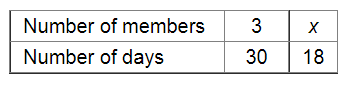# A group of 3 friends staying together, consume 54 kg of wheat every month.`
Question:

A group of 3 friends staying together, consume 54 kg of wheat every month. Some more friends join this group and they find that the same amount of wheat lasts for 18 days. How many new members are there in this group now?

Solution:

Let x be the number of new members in the group.Since more members can finish the wheat in less number of days, it is a case of inverse variation. Therefore, we get:

$3 \times 30=x \times 18$

$\Rightarrow 90=18 x$

$\Rightarrow x=\frac{90}{18}$

Thus, the number of new members in the group $=5-3=2$